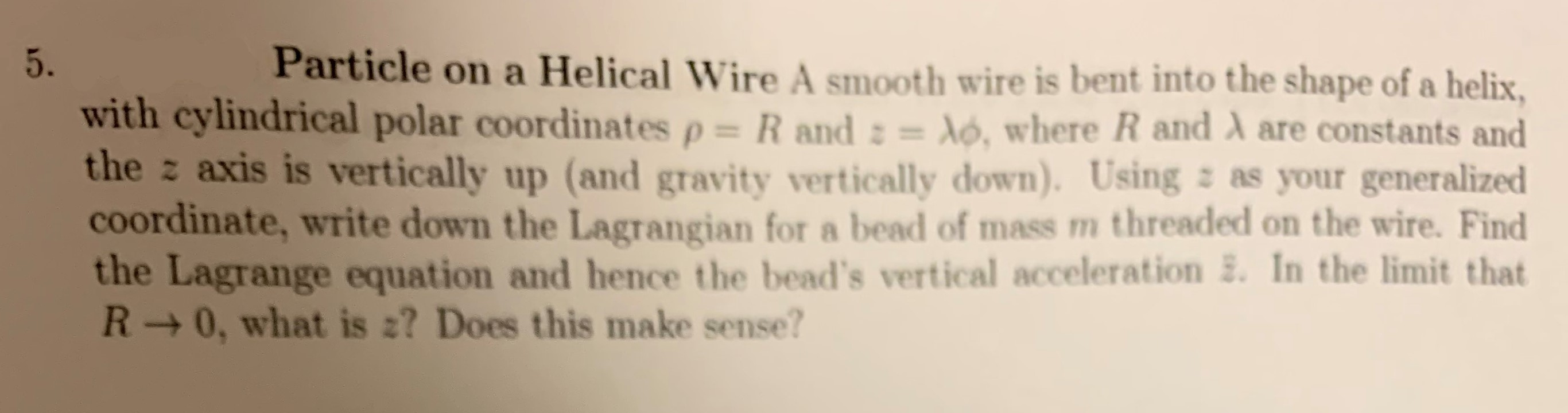# Particle on a Helical Wire A smooth wire is bent into the shape of a helix 5. with cylindrical polar coordinates p= R and : = dó, where R and A are constants and the z axis is vertically up (and gravity vertically down). Using z as your generalized coordinate, write down the Lagrangian for a bead of mass m threaded on the wire. Find the Lagrange equation and hence the bead's vertical acceleration . In the limit that R 0, what is 2? Does this make sense?

Questionhelp_outlineImage TranscriptioncloseParticle on a Helical Wire A smooth wire is bent into the shape of a helix 5. with cylindrical polar coordinates p= R and : = dó, where R and A are constants and the z axis is vertically up (and gravity vertically down). Using z as your generalized coordinate, write down the Lagrangian for a bead of mass m threaded on the wire. Find the Lagrange equation and hence the bead's vertical acceleration . In the limit that R 0, what is 2? Does this make sense? fullscreen

### Want to see the step-by-step answer?